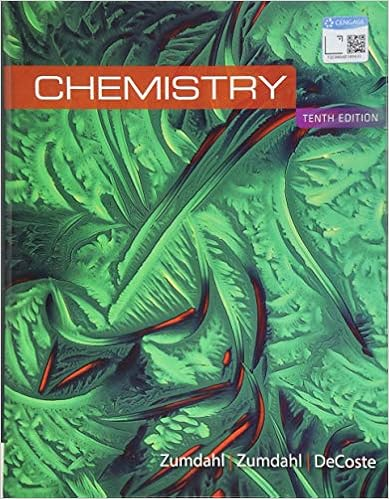# Be measured out in precise mass amounts it is easily

• No School
• NONE 0
• Lab Report
• 21
• 40% (5) 2 out of 5 people found this document helpful

This preview shows page 8 - 11 out of 21 pages.

##### We have textbook solutions for you!
The document you are viewing contains questions related to this textbook.The document you are viewing contains questions related to this textbook.
Chapter 15 / Exercise 9
Chemistry
ZumdahlExpert Verified
be measured out in precise mass amounts; it is easily obtained in a high state of purity and is soluble in water, thus making it an ideal substance to use to standardize a solution of NaOH. The results of a KHP titration with NaOH is shown in figure 2 below.
##### We have textbook solutions for you!
The document you are viewing contains questions related to this textbook.The document you are viewing contains questions related to this textbook.
Chapter 15 / Exercise 9
Chemistry
ZumdahlExpert Verified
CH204 Experiment 5: Strong Acid/Strong Base Titration spring 2014 Figure 2. The titration curve of KHP titrated with NaOH. The KHP in this titration is a weak acid. The equivalence point in a titration of a weak acid with a strong base is greater than pH=7 as a result of the hydrolysis of the strong conjugate base that is produced from the soluble salt. We will cover this concept in greater detail in experiment 7. The Method of Titration Example: We will perform a strong acid – strong base titration with an indicator. We want to determine the concentration of a sulfuric acid solution using a standardized sodium hydroxide solution and an indicator as our method of determining the amount (moles) of unknown acid or base that was neutralized during the procedure. The reaction stoichiometry is: H 2 SO 4 (aq) + 2NaOH (aq) → 2H 2 O (l) + Na 2 SO 4 (aq) We have: 1. A burette that holds a volume of 50.0 mL and is graduated in 0.01 mL increments. 2. Phenolphthalein, an indicator that is colorless in acidic solutions but is pink in basic solutions. 3. A standardized solution of 1.10 M sodium hydroxide solution. The procedure: 1. We fill the graduated cylinder to the 5.22 mL mark with our 1.10 M sodium hydroxide standard solution . 2. We will use a few drops of phenolphthalein as our indicator. Phenolphthalein is colorless in acidic solutions but will turn very light pink after the acid has been neutralized. 3. We will take a 50.0 mL sample of our unknown concentration sulfuric acid in a beaker and place it under the tip of the burette.
CH204 Experiment 5: Strong Acid/Strong Base Titration spring 2014 4. We begin adding the basic secondary standard, NaOH, until our acid sample solution turns very light pink, the end point . The point at which the indicator changes color and the titration is stopped. Theoretically, the sample volume of the sulfuric acid will still be colorless at exactly to the point in which the numbers of moles H + have been completely neutralized by the OH ions of our standard, the equivalence point . The equivalence point occurs when all the H + ions from the acid and OH ions from the base have been used up in the reaction. As a result, the solution is neutral; the pH = 7. Note: The equivalence point and the end point do not necessarily coincide. Therefore, the equivalence point and the end point are separate terms with different meanings. If we add more OH ions than are needed to exactly neutralize the H + ions in the sample acid, then the solution becomes basic and the color changes to pink. We want to add the sodium hydroxide until the solution is very slightly pink (the end point). Ideally, the end point will equal the equivalence point. However, when the color change of the indicator becomes visible, the [OH - ] is
•••